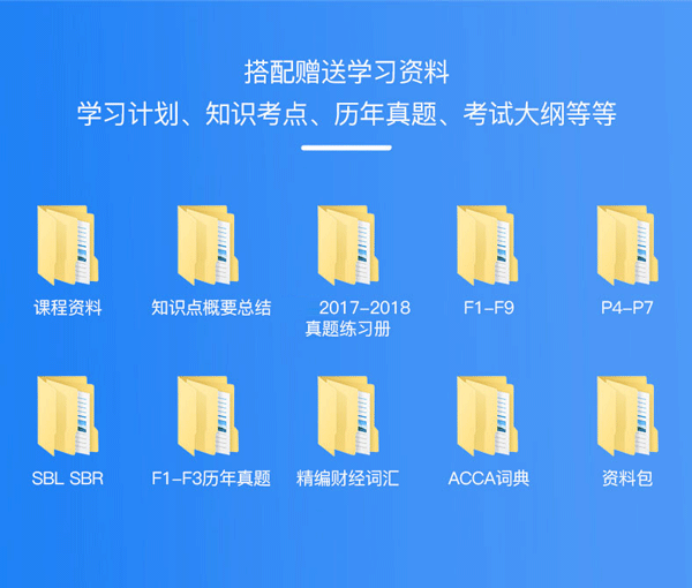# ACCA考试Economic Order Quantity怎么学？

2020年新版ACCA备考资料下载
• 考纲对比
• 学习计划
• 思维导图
• 复习资料
• 历年真题
• 词典及公式

ACCA考试的内容是由易到难的过程，学员要想成功的通过所有的考试，需要一门一门课程的学习才可以。ACCA考试知识点较多，ACCA考试Economic Order Quantity怎么学？1．The economic order quantity(EOQ) is the order quantity which minimizes inventory costs.The EOQ can be calculated using a table,graph or formula.

2．Economic order theory assumes that the average inventory held is equal to one half of the reorder quantity.

3．Example:

Suppose a company purchases raw material at a cost of \$16 per unit.The annual demand for the raw material is 25,000 units,The holding cost per unit is \$6.40 and the cost of placing an order is \$32.

We can tabulate the annual relevant costs for various order quantities as follows.Notes

(1)Average inventory＝Order quantity÷2(ie assuming no safety inventory)

(2)Number of orders＝annual demand÷order quantity

(3)Annual holding cost＝Average inventory×\$6.40

(4)Annual order cost＝Number of orders×\$32

You will see that the economic order quantity is 500 units,At this point the total annual relevant costs are at a minimum.

ACCA考试，ACCA会员，ACCA成员